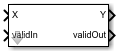Divide by Constant HDL Optimized

Divide input by a constant and round to integer and generate optimized HDL code

• Library:
• Fixed-Point Designer HDL Support / Math Operations

•Description

The Divide by Constant HDL Optimized block outputs the result of dividing the input by a constant and rounds the result to an integer using the specified rounding method using an HDL-optimized architecture with cycle-true latency.

The Divide by Constant HDL Optimized block uses an algorithm that is functionally similar to the Granlund-Montgomery-Warren Method. The division operation is computed via a multiplication by inverse, which generally results in better performance on embedded systems.

Ports

Input

expand all

Dividend, specified as a real scalar.

Data Types: single | double | int8 | int16 | int32 | uint8 | uint16 | uint32 | Boolean | fixed point

Whether input is valid, specified as a Boolean scalar. This control signal indicates when the data from the X input port is valid. When this value is 1 (true), the block captures the value on the X input port. When this value is 0 (false), the block ignores the input samples.

Data Types: Boolean

Output

expand all

Result of division and round operation, returned as a scalar.

Data Types: single | double | int8 | int16 | int32 | uint8 | uint16 | uint32 | Boolean | fixed point

Whether the output data is valid, returned as a Boolean scalar. When the value of this control signal is 1 (true), the block has successfully computed the output Y. When this value is 0 (false), the output data is not valid.

Data Types: Boolean

Parameters

expand all

Divisor, specified as a positive, real-valued, finite scalar.

Programmatic Use

 Block Parameter: Denominator Type: character vector Values: MATLAB® expression that evaluates to a positive, real-valued, finite fixed point or numeric value Default: '10'

Data Types: single | double | int8 | int16 | int32 | int64 | uint8 | uint16 | uint32 | uint64 | Boolean | fixed point

Rounding method to use, specified as one of these values:

• Floor — Round to nearest integer in the direction of negative infinity.

• Ceiling — Round to nearest integer in the direction of positive infinity.

• Nearest — Round to the nearest integer. Ties are rounded to the nearest integer in the direction of positive infinity.

• Zero — Round to the nearest integer in the direction of zero.

• Convergent — Round to the nearest integer. Ties are rounded to the nearest even integer.

Programmatic Use

 Block Parameter: RndMeth Type: character vector Values: 'Floor' | 'Ceiling' | 'Nearest' | 'Zero' | 'Convergent' Default: 'Floor'

Tips

The blocks Divide by Constant HDL Optimized, Real Divide HDL Optimized, and Complex Divide HDL Optimized all perform the division operation and generate optimized HDL code.

• Real Divide HDL Optimized and Complex Divide HDL Optimized are based on a CORIDC algorithm. These blocks accept a wide variety of inputs, but will result in greater latency.

• Divide by Constant HDL Optimized accepts only real inputs and a constant divisor. Use of this block consumes DSP slices, but will complete the division operation in fewer cycles and at a higher clock rate.

Algorithms

The Divide by Constant HDL Optimized uses an HDL-optimized architecture with cycle-true latency.

The Divide by Constant HDL Optimized block uses an algorithm that is functionally similar to the Granlund-Montgomery-Warren Method. The division operation is computed via a multiplication by inverse, which generally results in better performance on embedded systems.

Extended Capabilities